Eric Smith

Graphing Inequalities in Two Variables

Slide Duration:

Section 1: Properties of Real Numbers
Basic Types of Numbers

30m 41s

Intro
0:00
Objectives
0:07
Basic Types of Numbers
0:36
Natural Numbers
1:02
Whole Numbers
1:29
Integers
2:04
Rational Numbers
2:38
Irrational Numbers
5:06
Imaginary Numbers
6:48
Basic Types of Numbers Cont.
8:09
The Big Picture
8:10
Real vs. Imaginary Numbers
8:30
Rational vs. Irrational Numbers
8:48
Basic Types of Numbers Cont.
10:55
Number Line
11:06
Absolute Value
11:44
Inequalities
12:39
Example 1
13:16
Example 2
17:30
Example 3
21:56
Example 4
24:27
Example 5
27:48
Operations on Numbers

19m 26s

Intro
0:00
Objectives
0:06
Operations on Numbers
0:25
0:53
Subtraction
1:33
Multiplication & Division
2:19
Exponents
3:24
Bases
4:04
Square Roots
4:59
Principle Square Roots
5:09
Perfect Squares
6:32
Simplifying and Combining Roots
6:52
Example 1
8:16
Example 2
12:30
Example 3
14:02
Example 4
16:27
Order of Operations

12m 6s

Intro
0:00
Objectives
0:06
The Order of Operations
0:25
Work Inside Parentheses
0:42
Simplify Exponents
0:52
Multiplication & Division from Left to Right
0:57
Addition & Subtraction from Left to Right
1:11
Remember PEMDAS
1:21
The Order of Operations Cont.
2:27
Example
2:43
Example 1
3:55
Example 2
5:36
Example 3
7:35
Example 4
8:56
Properties of Real Numbers

18m 52s

Intro
0:00
Objectives
0:07
The Properties of Real Numbers
0:23
Commutative Property of Addition and Multiplication
0:44
Associative Property of Addition and Multiplication
1:50
Distributive Property of Multiplication Over Addition
3:20
Division Property of Zero
4:46
Division Property of One
5:23
Multiplication Property of Zero
5:56
Multiplication Property of One
6:17
6:29
Why Are These Properties Important?
6:53
Example 1
9:16
Example 2
13:04
Example 3
14:30
Example 4
16:57
Section 2: Linear Equations
The Vocabulary of Linear Equations

12m 22s

Intro
0:00
Objectives
0:09
The Vocabulary of Linear Equations
0:44
Variables
0:52
Terms
1:09
Coefficients
1:40
Like Terms
2:18
Examples of Like Terms
2:37
Expressions
4:01
Equations
4:26
Linear Equations
5:04
Solutions
5:55
Example 1
6:16
Example 2
7:16
Example 3
8:45
Example 4
10:20
Solving Linear Equations in One Variable

28m 52s

Intro
0:00
Objectives
0:08
Solving Linear Equations in One Variable
0:34
Conditional Cases
0:51
Identity Cases
1:09
1:30
Solving Linear Equations in One Variable Cont.
2:00
2:10
Multiplication Property of Equality
2:43
Steps to Solve Linear Equations
3:14
Example 1
4:22
Example 2
8:21
Example 3
12:32
Example 4
14:19
Example 5
17:25
Example 6
22:17
Solving Formulas

12m 2s

Intro
0:00
Objectives
0:06
Solving Formulas
0:18
Formulas
0:26
Use the Same Properties as Solving Linear Equations
1:36
1:55
Multiplication Property of Equality
1:58
Steps to Solve Formulas
2:43
Example 1
3:56
Example 2
6:09
Example 3
8:39
Applications of Linear Equations

28m 41s

Intro
0:00
Objectives
0:10
Applications of Linear Equations
0:43
The Six-Step Method to Solving Word Problems
0:55
Common Terms
3:12
Example 1
5:03
Example 2
9:40
Example 3
13:48
Example 4
17:58
Example 5
23:28
Applications of Linear Equations, Motion & Mixtures

24m 26s

Intro
0:00
Objectives
0:21
Motion and Mixtures
0:46
Motion Problems: Distance, Rate, and Time
1:06
Mixture Problems: Amount, Percent, and Total
1:27
The Table Method
1:58
The Beaker Method
3:38
Example 1
5:05
Example 2
9:44
Example 3
14:20
Example 4
19:13
Section 3: Graphing
Rectangular Coordinate System

22m 55s

Intro
0:00
Objectives
0:11
The Rectangular Coordinate System
0:39
The Cartesian Coordinate System
0:40
X-Axis
0:54
Y-Axis
1:04
Origin
1:11
1:26
Ordered Pairs
2:10
Example 1
2:55
The Rectangular Coordinate System Cont.
6:09
X-Intercept
6:45
Y-Intercept
6:55
Relation of X-Values and Y-Values
7:30
Example 2
11:03
Example 3
12:13
Example 4
14:10
Example 5
18:38
Slope & Graphing

27m 58s

Intro
0:00
Objectives
0:11
Slope and Graphing
0:48
Standard Form
1:14
Example 1
2:24
Slope and Graphing Cont.
4:58
Slope, m
5:07
Slope is Rise over Run
6:11
Don't Mix Up the Coordinates
8:20
Example 2
9:39
Slope and Graphing Cont.
14:26
Slope-Intercept Form
14:34
Example 3
16:55
Example 4
18:00
Slope and Graphing Cont.
19:00
Rewriting an Equation in Slope-Intercept Form
19:39
Rewriting an Equation in Standard Form
20:09
Slopes of Vertical & Horizontal Lines
20:56
Example 5
22:49
Example 6
24:09
Example 7
25:59
Example 8
26:57
Linear Equations in Two Variables

20m 36s

Intro
0:00
Objectives
0:13
Linear Equations in Two Variables
0:36
Point-Slope Form
1:07
Substitute in the Point and the Slope
2:21
Parallel Lines: Two Lines with the Same Slope
4:05
Perpendicular Lines: Slopes are Negative Reciprocals of Each Other
4:39
Perpendicular Lines: Product of Slopes is -1
5:24
Example 1
6:02
Example 2
7:50
Example 3
10:49
Example 4
13:26
Example 5
15:30
Example 6
17:43
Section 4: Functions
Introduction to Functions

21m 24s

Intro
0:00
Objectives
0:07
Introduction to Functions
0:58
Relations
1:03
Functions
1:37
Independent Variables
2:00
Dependent Variables
2:11
Function Notation
2:21
Function
3:43
Input and Output
3:53
Introduction to Functions Cont.
4:45
Domain
4:46
Range
4:55
Functions Represented by a Diagram
6:41
Natural Domain
9:11
Evaluating Functions
12:02
Example 1
13:13
Example 2
15:03
Example 3
16:18
Example 4
19:54
Graphing Functions

16m 12s

Intro
0:00
Objectives
0:09
Graphing Functions
0:54
Using Slope-Intercept Form
1:56
Vertical Line Test
2:58
Determining the Domain
4:20
Determining the Range
5:43
Example 1
6:06
Example 2
7:18
Example 3
8:31
Example 4
11:04
Section 5: Systems of Linear Equations
Systems of Linear Equations

25m 54s

Intro
0:00
Objectives
0:13
Systems of Linear Equations
0:46
System of Equations
0:51
System of Linear Equations
1:15
Solutions
1:35
Points as Solutions
1:53
Finding Solutions Graphically
5:13
Example 1
6:37
Example 2
12:07
Systems of Linear Equations Cont.
17:01
One Solution, No Solution, or Infinite Solutions
17:10
Example 3
18:31
Example 4
22:37
Solving a System Using Substitution

20m 1s

Intro
0:00
Objectives
0:09
Solving a System Using Substitution
0:32
Substitution Method
1:24
Substitution Example
2:35
One Solution, No Solution, or Infinite Solutions
7:50
Example 1
9:45
Example 2
12:48
Example 3
15:01
Example 4
17:30
Solving a System Using Elimination

19m 40s

Intro
0:00
Objectives
0:09
Solving a System Using Elimination
0:27
Elimination Method
0:42
Elimination Example
2:01
One Solution, No Solution, or Infinite Solutions
7:05
Example 1
8:53
Example 2
11:46
Example 3
15:37
Example 4
17:45
Applications of Systems of Equations

24m 34s

Intro
0:00
Objectives
0:12
Applications of Systems of Equations
0:30
Word Problems
1:31
Example 1
2:17
Example 2
7:55
Example 3
13:07
Example 4
17:15
Section 6: Inequalities
Solving Linear Inequalities in One Variable

17m 13s

Intro
0:00
Objectives
0:08
Solving Linear Inequalities in One Variable
0:37
Inequality Expressions
0:46
Linear Inequality Solution Notations
3:40
Inequalities
3:51
Interval Notation
4:04
Number Lines
4:43
Set Builder Notation
5:24
Use Same Techniques as Solving Equations
6:59
'Flip' the Sign when Multiplying or Dividing by a Negative Number
7:12
'Flip' Example
7:50
Example 1
8:54
Example 2
11:40
Example 3
14:01
Compound Inequalities

16m 13s

Intro
0:00
Objectives
0:07
Compound Inequalities
0:37
'And' vs. 'Or'
0:44
'And'
3:24
'Or'
3:35
'And' Symbol, or Intersection
3:51
'Or' Symbol, or Union
4:13
Inequalities
4:41
Example 1
6:22
Example 2
9:30
Example 3
11:27
Example 4
13:49
Solving Equations with Absolute Values

14m 12s

Intro
0:00
Objectives
0:08
Solve Equations with Absolute Values
0:18
Solve Equations with Absolute Values Cont.
1:11
Steps to Solving Equations with Absolute Values
2:21
Example 1
3:23
Example 2
6:34
Example 3
10:12
Inequalities with Absolute Values

17m 7s

Intro
0:00
Objectives
0:07
Inequalities with Absolute Values
0:23
Recall…
2:08
Example 1
3:39
Example 2
6:06
Example 3
8:14
Example 4
10:29
Example 5
13:29
Graphing Inequalities in Two Variables

15m 33s

Intro
0:00
Objectives
0:07
Graphing Inequalities in Two Variables
0:32
Split Graph into Two Regions
1:53
Graphing Inequalities
5:44
Test Points
6:20
Example 1
7:11
Example 2
10:17
Example 3
13:06
Systems of Inequalities

21m 13s

Intro
0:00
Objectives
0:08
Systems of Inequalities
0:24
Test Points
1:10
Steps to Solve Systems of Inequalities
1:25
Example 1
2:23
Example 2
7:28
Example 3
12:51
Section 7: Polynomials
Integer Exponents

44m 51s

Intro
0:00
Objectives
0:09
Integer Exponents
0:42
Exponents 'Package' Multiplication
1:25
Example 1
2:00
Example 2
3:13
Integer Exponents Cont.
4:50
Product Rule for Exponents
4:51
Example 3
7:16
Example 4
10:15
Integer Exponents Cont.
13:13
Power Rule for Exponents
13:14
Power Rule with Multiplication and Division
15:33
Example 5
16:18
Integer Exponents Cont.
20:04
Example 6
20:41
Integer Exponents Cont.
25:52
Zero Exponent Rule
25:53
Quotient Rule
28:24
Negative Exponents
30:14
Negative Exponent Rule
32:27
Example 7
34:05
Example 8
36:15
Example 9
39:33
Example 10
43:16

18m 33s

Intro
0:00
Objectives
0:07
0:25
Terms
0:33
Coefficients
0:51
1:13
Like Terms
1:29
Polynomials
2:21
Monomials, Binomials, Trinomials, and Polynomials
5:41
Degrees
7:00
Evaluating Polynomials
8:12
9:25
Example 1
11:48
Example 2
13:00
Example 3
14:41
Example 4
16:15
Multiplying Polynomials

25m 7s

Intro
0:00
Objectives
0:06
Multiplying Polynomials
0:41
Distributive Property
1:00
Example 1
2:49
Multiplying Polynomials Cont.
8:22
Organize Terms with a Table
8:23
Example 2
13:40
Multiplying Polynomials Cont.
16:33
Multiplying Binomials with FOIL
16:48
Example 3
18:49
Example 4
20:04
Example 5
21:42
Dividing Polynomials

44m 56s

Intro
0:00
Objectives
0:07
Dividing Polynomials
0:29
Dividing Polynomials by Monomials
2:10
Dividing Polynomials by Polynomials
2:59
Dividing Numbers
4:09
Dividing Polynomials Example
8:39
Example 1
12:35
Example 2
14:40
Example 3
16:45
Example 4
21:13
Example 5
24:33
Example 6
29:02
Dividing Polynomials with Synthetic Division Method
33:36
Example 7
38:43
Example 8
42:24
Section 8: Factoring Polynomials
Greatest Common Factor & Factor by Grouping

28m 27s

Intro
0:00
Objectives
0:09
Greatest Common Factor
0:31
Factoring
0:40
Greatest Common Factor (GCF)
1:48
GCF for Polynomials
3:28
Factoring Polynomials
6:45
Prime
8:21
Example 1
9:14
Factor by Grouping
14:30
Steps to Factor by Grouping
17:03
Example 2
17:43
Example 3
19:20
Example 4
20:41
Example 5
22:29
Example 6
26:11
Factoring Trinomials

21m 44s

Intro
0:00
Objectives
0:06
Factoring Trinomials
0:25
Recall FOIL
0:26
Factor a Trinomial by Reversing FOIL
1:52
Tips when Using Reverse FOIL
5:31
Example 1
7:04
Example 2
9:09
Example 3
11:15
Example 4
13:41
Factoring Trinomials Cont.
15:50
Example 5
18:42
Factoring Trinomials Using the AC Method

30m 9s

Intro
0:00
Objectives
0:08
Factoring Trinomials Using the AC Method
0:27
Factoring when Leading Term has Coefficient Other Than 1
1:07
Reversing FOIL
1:18
Example 1
1:46
Example 2
4:28
Factoring Trinomials Using the AC Method Cont.
7:45
The AC Method
8:03
Steps to Using the AC Method
8:19
Tips on Using the AC Method
9:29
Example 3
10:45
Example 4
16:50
Example 5
21:08
Example 6
24:58
Special Factoring Techniques

30m 14s

Intro
0:00
Objectives
0:07
Special Factoring Techniques
0:26
Difference of Squares
1:46
Perfect Square Trinomials
2:38
No Sum of Squares
3:32
Special Factoring Techniques Cont.
4:03
Difference of Squares Example
4:04
Perfect Square Trinomials Example
5:29
Example 1
7:31
Example 2
9:59
Example 3
11:47
Example 4
15:09
Special Factoring Techniques Cont.
19:07
Sum of Cubes and Difference of Cubes
19:08
Example 5
23:13
Example 6
26:12

23m 38s

Intro
0:00
Objectives
0:08
0:19
0:20
Zero Factor Property
1:39
Zero Factor Property Example
2:34
Example 1
4:00
Solving Quadratic Equations by Factoring Cont.
5:54
Example 2
7:28
Example 3
11:09
Example 4
14:22
Solving Quadratic Equations by Factoring Cont.
18:17
Higher Degree Polynomial Equations
18:18
Example 5
20:22

29m 27s

Intro
0:00
Objectives
0:12
0:29
Linear Factors
0:38
1:22
Principle of Square Roots
3:36
Completing the Square
4:50
Steps for Using Completing the Square
5:15
Completing the Square Works on All Quadratic Equations
6:41
7:28
Discriminants
8:25
10:11
Example 1
11:54
Example 2
13:03
Example 3
16:30
Example 4
21:29
Example 5
25:07

16m 47s

Intro
0:00
Objectives
0:08
0:24
Using a Substitution
0:53
U-Substitution
1:26
Example 1
2:07
Example 2
5:36
Example 3
8:31
Example 4
11:14

29m 4s

Intro
0:00
Objectives
0:09
0:35
Squared Variable
0:40
Principle of Square Roots
0:51
Example 1
1:09
Example 2
2:04
3:34
Example 3
4:42
Example 4
13:33
Example 5
20:50

26m 53s

Intro
0:00
Objectives
0:06
0:39
Axis of Symmetry
1:46
Vertex
2:12
Transformations
2:57
3:23
Example 1
5:06
Example 2
6:02
Example 3
9:07
11:26
Completing the Square
12:02
Vertex Shortcut
12:16
Example 4
13:49
Example 5
17:25
Example 6
20:07
Example 7
23:43
Polynomial Inequalities

21m 42s

Intro
0:00
Objectives
0:07
Polynomial Inequalities
0:30
Solving Polynomial Inequalities
1:20
Example 1
2:45
Polynomial Inequalities Cont.
5:12
Larger Polynomials
5:13
Positive or Negative Intervals
7:16
Example 2
9:01
Example 3
13:53
Section 10: Rational Equations
Multiply & Divide Rational Expressions

26m 41s

Intro
0:00
Objectives
0:09
Multiply and Divide Rational Expressions
0:44
Rational Numbers
0:55
Dividing by Zero
1:45
Canceling Extra Factors
2:43
Negative Signs in Fractions
4:52
Multiplying Fractions
6:26
Dividing Fractions
7:17
Example 1
8:04
Example 2
14:01
Example 3
16:23
Example 4
18:56
Example 5
22:43

20m 24s

Intro
0:00
Objectives
0:07
0:41
Common Denominators
0:52
Common Denominator Examples
1:14
Steps to Adding and Subtracting Rational Expressions
2:39
Example 1
3:34
Example 2
5:27
Adding and Subtracting Rational Expressions Cont.
6:57
Least Common Denominators
6:58
Transitioning from Fractions to Rational Expressions
9:08
Identifying Least Common Denominators for Rational Expressions
9:56
10:41
Example 3
11:19
Example 4
12:36
Example 5
15:08
Example 6
16:46
Complex Fractions

18m 23s

Intro
0:00
Objectives
0:09
Complex Fractions
0:37
Dividing to Simplify Complex Fractions
1:10
Example 1
2:03
Example 2
3:58
Complex Fractions Cont.
9:15
Using the Least Common Denominator to Simplify Complex Fractions
9:16
10:07
Example 3
10:42
Example 4
14:28
Solving Rational Equations

16m 24s

Intro
0:00
Objectives
0:07
Solving Rational Equations
0:23
Isolate the Specified Variable
1:23
Example 1
1:58
Example 2
5:00
Example 3
8:23
Example 4
13:25
Rational Inequalities

18m 54s

Intro
0:00
Objectives
0:06
Rational Inequalities
0:18
Testing Intervals for Rational Inequalities
0:38
Steps to Solving Rational Inequalities
1:05
Tips to Solving Rational Inequalities
2:27
Example 1
3:33
Example 2
12:21
Applications of Rational Expressions

20m 20s

Intro
0:00
Objectives
0:07
Applications of Rational Expressions
0:27
Work Problems
1:05
Example 1
2:58
Example 2
6:45
Example 3
13:17
Example 4
16:37
Variation & Proportion

27m 4s

Intro
0:00
Objectives
0:10
Variation and Proportion
0:34
Variation
0:35
Inverse Variation
1:01
Direct Variation
1:10
Setting Up Proportions
1:31
Example 1
2:27
Example 2
5:36
Variation and Proportion Cont.
8:29
Inverse Variation
8:30
Example 3
9:20
Variation and Proportion Cont.
12:41
Constant of Proportionality
12:42
Example 4
13:59
Variation and Proportion Cont.
16:17
Varies Directly as the nth Power
16:30
Varies Inversely as the nth Power
16:53
Varies Jointly
17:09
Combining Variation Models
17:36
Example 5
19:09
Example 6
22:10
Rational Exponents

14m 32s

Intro
0:00
Objectives
0:07
Rational Exponents
0:32
Power on Top, Root on Bottom
1:05
Example 1
1:37
Rational Exponents Cont.
4:04
Using Rules from Exponents for Radicals as Exponents
4:05
Combining Terms Under a Single Root
4:50
Example 2
5:21
Example 3
7:39
Example 4
11:23
Example 5
13:14
Simplify Rational Exponents

15m 12s

Intro
0:00
Objectives
0:07
Simplify Rational Exponents
0:25
0:26
Product Rule to Simplify Square Roots
1:11
1:42
Applications of Product and Quotient Rules
2:17
Higher Roots
2:48
Example 1
3:39
Example 2
6:35
Example 3
8:41
Example 4
11:09

17m 22s

Intro
0:00
Objectives
0:07
0:33
Like Terms
1:29
Bases and Exponents May be Different
2:02
Bases and Powers Must be Same when Adding and Subtracting
2:42
3:55
Example 1
4:47
Example 2
6:00
7:10
Simplify the Bases to Look the Same
7:25
Example 3
8:23
Example 4
11:45
Example 5
15:10

19m 24s

Intro
0:00
Objectives
0:08
0:25
0:26
1:11
Don’t Distribute Powers
2:54
4:25
Rationalizing Denominators
6:40
Example 1
7:22
Example 2
8:32
9:23
Rationalizing Denominators with Higher Roots
9:25
Example 3
10:51
Example 4
11:53
13:13
Rationalizing Denominators with Conjugates
13:14
Example 5
15:52
Example 6
17:25

15m 5s

Intro
0:00
Objectives
0:07
0:17
0:18
Isolate the Roots and Raise to Power
0:34
Example 1
1:13
Example 2
3:09
7:04
7:05
Example 3
7:54
Example 4
13:07
Complex Numbers

29m 16s

Intro
0:00
Objectives
0:06
Complex Numbers
1:05
Imaginary Numbers
1:08
Complex Numbers
2:27
Real Parts
2:48
Imaginary Parts
2:51
Commutative, Associative, and Distributive Properties
3:35
4:04
Multiplying Complex Numbers
6:16
Dividing Complex Numbers
8:59
Complex Conjugate
9:07
Simplifying Powers of i
14:34
Shortcut for Simplifying Powers of i
18:33
Example 1
21:14
Example 2
22:15
Example 3
23:38
Example 4
26:33
Bookmark & Share Embed

## Copy & Paste this embed code into your website’s HTML

Please ensure that your website editor is in text mode when you paste the code.
(In Wordpress, the mode button is on the top right corner.)
×
• - Allow users to view the embedded video in full-size.
Since this lesson is not free, only the preview will appear on your website.

• ## Related Books2 answersLast reply by: Sylvia WangThu Apr 2, 2020 9:22 AMPost by Sylvia Wang on April 1, 2020is there a possibility that there wouldn't be a solution?1 answerLast reply by: Professor Eric SmithWed Sep 3, 2014 1:36 PMPost by sherman boey on August 13, 2014for example 1 u did not use dotted line1 answerLast reply by: Professor Eric SmithSun Jul 6, 2014 2:36 PMPost by patrick guerin on July 6, 2014Thank you for the lecture!

### Graphing Inequalities in Two Variables

• To graph an inequality in two variables begin by graphing the corresponding line. It should be dotted if the inequality is strict, and solid if the inequality has “or equal.”
• To graph the line, remember you can use slope-intercept form with the y-intercept and the slope to graph it quickly.
• Shade on one side of the line, or the other. A test point can be used to determine which side of the line should be shaded.

### Graphing Inequalities in Two Variables

Graph y > x - 2
• Find slope and intercept
b = - 2
m = 1
• Determine line type
• Dashed since >
• Test point (0,0)
0 > 0 - 2
0 > - 2
Which is false
Graph utilizing properties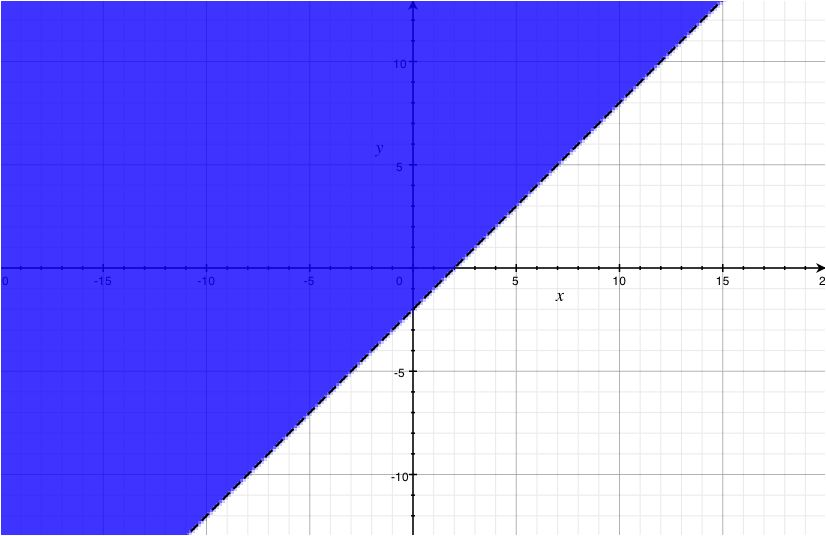Graph y < 2x
• Find slope and intercept
b = 0
m = 2
• Determine line type
Dashed since <
• Test point (1,0)
0 < 2(1)
0 < 2
Which is true
Graph utilizing properties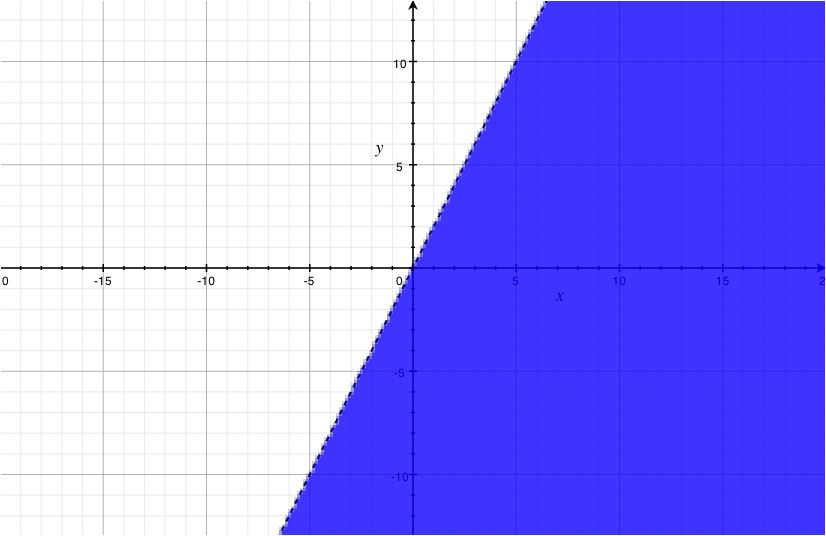Graph y ≥ 5x + 1
• Find slope and intercept
b = 5m = 1
• Determine line type
• Solid since ≥
• Test point (0,0)
0 ≥ 5(0) + 1
0 ≥ 1
Which is false
Graph utilizing properties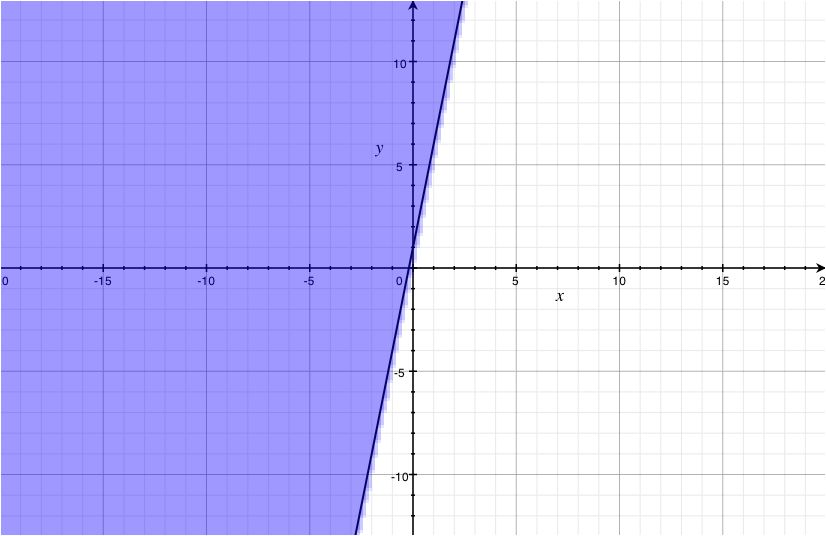Graph y ≥ [x/3] + 2
• Find slope and intercept
b = 2
m = [1/3]
• Determine line type
Solid since
• Test point (0,0)
0 ≥ [0/3] + 2
0 ≥ 2
Which is false
Graph utilizing properties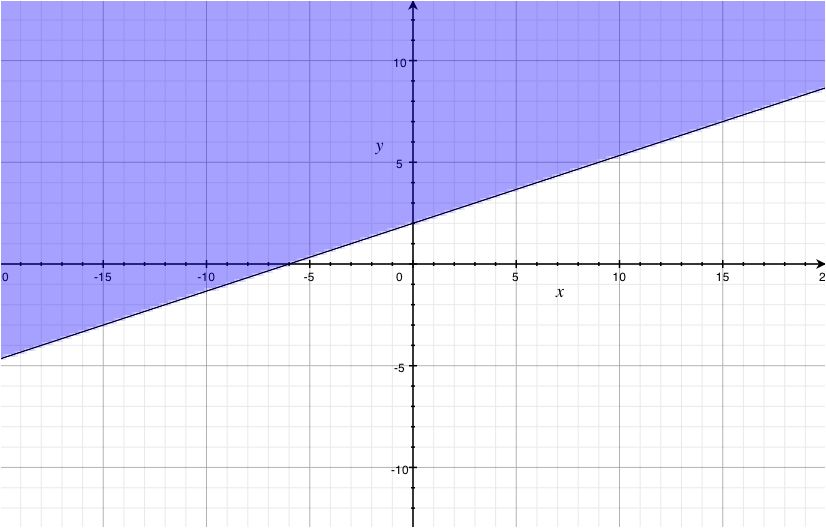Graph y ≤ x + 7
• Find slope and intercept
b = 7
m = 1
• Determine line type
• Solid since £
• Test point (0,0)
0 ≤ 0 + 7
0 ≤ 7
Which is true
Graph utilizing properties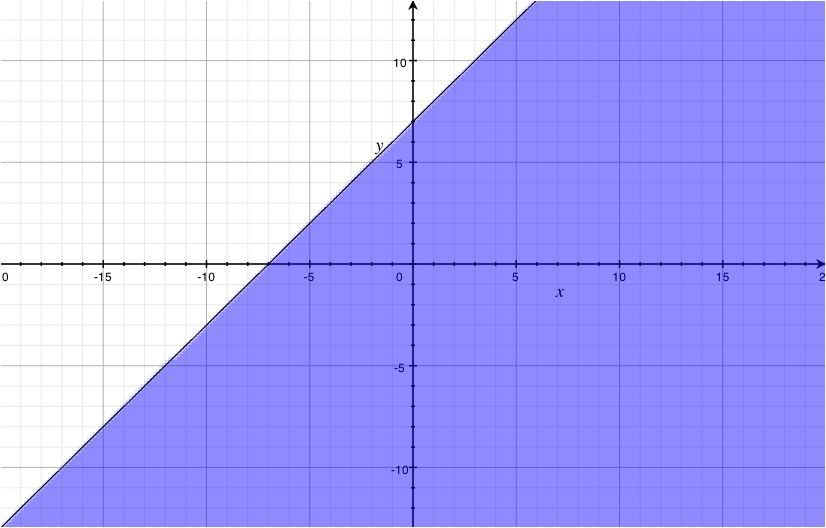Graph y − x > 5
• Find slope and intercept
b = 5
m = 1
• Determine line type
• Dashed since >
• Test point (0,0)
0 - 0 > 5
0 > 5
Which is false
Graph utilizing properties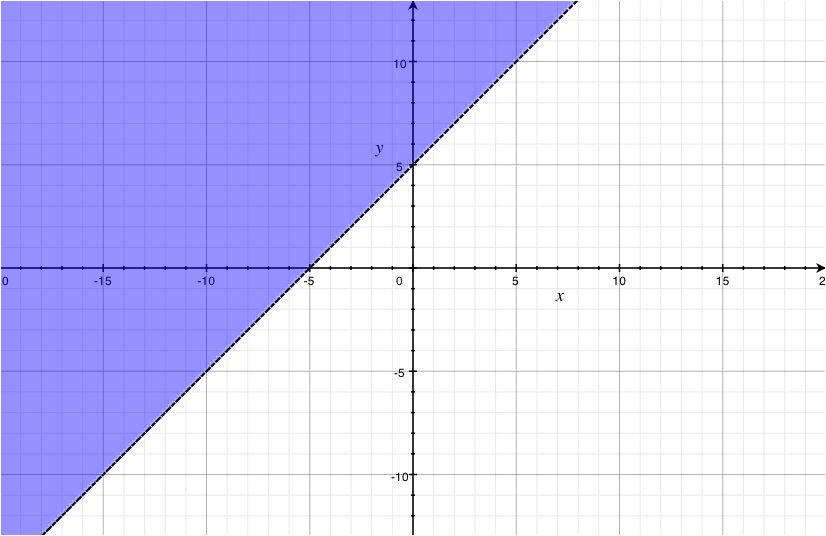Graph 2y + x <− 1
• Find slope and intercept
b = − 1
m = − [1/2]
• Determine line type
Dashed since <
• Test point (0,0)
2(0) + 0 < - 1
0 < - 1
Which is false
Graph utilizing properties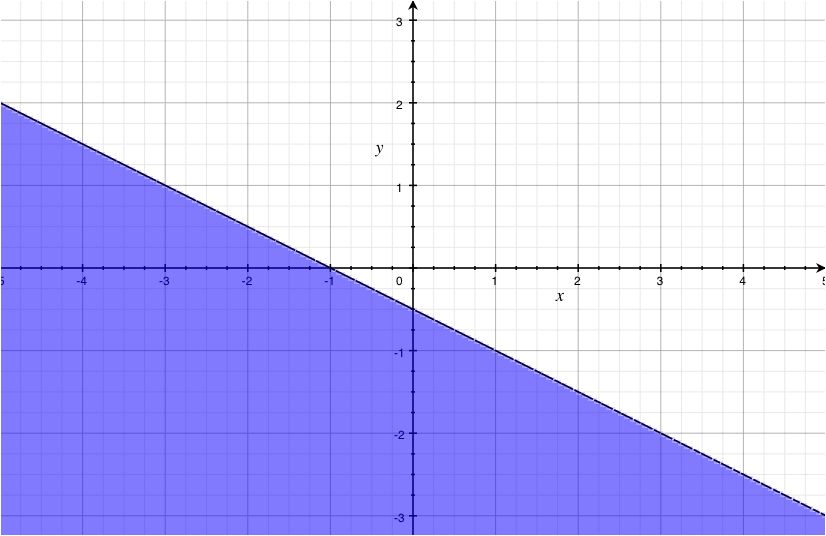Graph y + 2x > 3
• Find 2 points using intercepts
x = 0 → (0,3)y = 0 → (3,0)
• Determine line type
Dashed since >
• Test point (0,0)
0 + 2(0) > 3
0 > 3
Which is false
Graph utilizing properties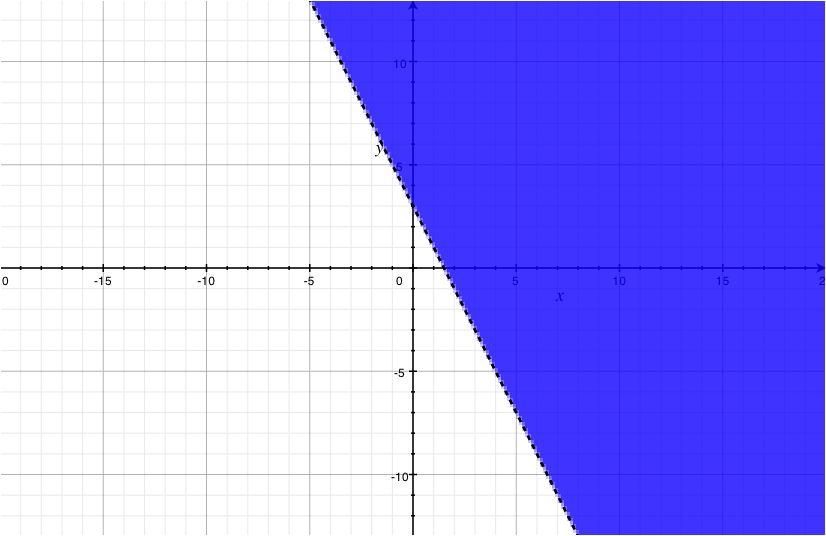Graph 3y - x > - 5
• Find 2 points using intercepts
x = 0 → (0, − [5/3])y = 0 → (5,0)
• Determine line type
Dashed since >
• Test point (0,0)
3(0) - 0 > - 5
0 > - 5
Which is true
Graph utilizing properties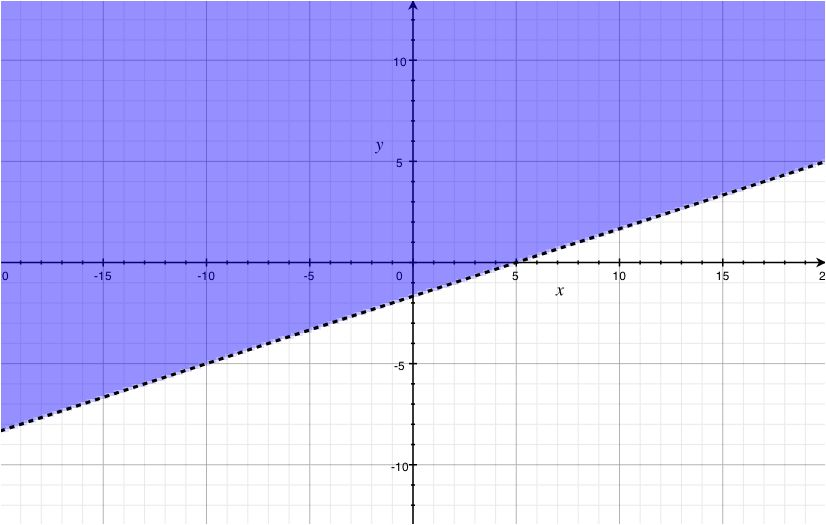Graph [(4y + 5x)/2] ≤ 2
• Find 2 points using intercepts
x = 0 → (0,1)y = 0 → ([4/5],0)
• Determine line type
Solid since ≤
• Test point (0,0)
[(4(0) + 5(0))/2] ≤ 2
[0/2] ≤ 2
0 ≤ 2
Which is true
Graph utilizing properties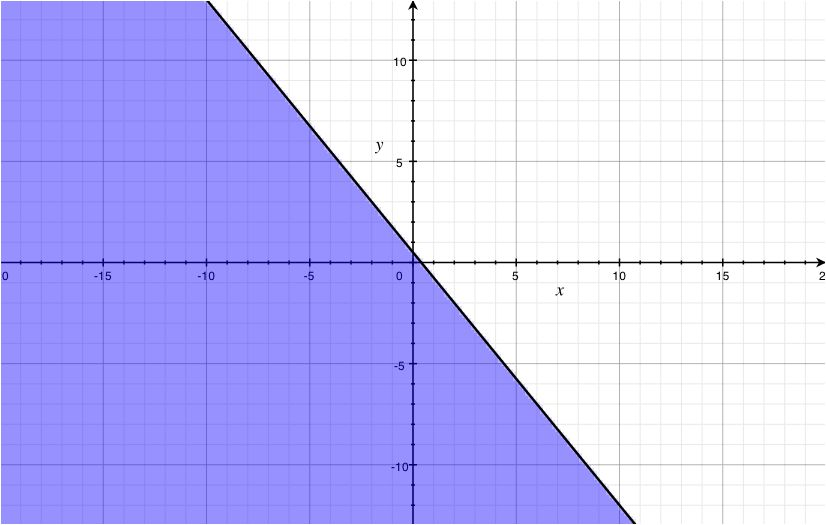*These practice questions are only helpful when you work on them offline on a piece of paper and then use the solution steps function to check your answer.

### Graphing Inequalities in Two Variables

Lecture Slides are screen-captured images of important points in the lecture. Students can download and print out these lecture slide images to do practice problems as well as take notes while watching the lecture.

• Intro 0:00
• Objectives 0:07
• Graphing Inequalities in Two Variables 0:32
• Split Graph into Two Regions
• Graphing Inequalities 5:44
• Test Points
• Example 1 7:11
• Example 2 10:17
• Example 3 13:06

### Transcription: Graphing Inequalities in Two Variables

Welcome back to www.educator.com.0000

In this lesson we are going to look at graphing inequalities using two variables.0002

In order to make this process work out , we will first briefly review how to just simply graph lines.0009

We will see how this works with our inequalities which involved entire regions of our graph.0016

The important parts for highlighting these regions will be shading in the proper part of the graph.0022

Watch for these things to come into play.0028

Recall it when you are dealing with any inequality it is not just usually an isolated point, it is usually a whole range of solutions.0035

When we are working with graphing, and we have more than one variable to worry about we are dealing with points like x and y.0043

If we are looking for the x and y that will make an inequality true, we are not talking about just one point on a graph,0052

we are actually talking about an entire region of the graph, and all the little points in that region.0058

The way we do this is when you start off by graphing the equation, corresponding with the inequality.0065

Let me show you what I mean by that.0071

I’m looking at the inequality y > or = 6/5x + 7 rather than trying to tackle on the entire inequality first,0074

I looked at the corresponding equation like y = 6/5x +7.0086

In doing so, I can look at my techniques for graphing just that equation.0093

Maybe find the slope and find the y intercept.0098

We will see how that equation connects back to our inequality.0106

What we are going to find is that equation is going to split our entire graph into two regions.0115

In one of the regions I will involve our solution and the other region will not.0121

I have cooked up another example, so you can see how this works.0126

We are going to start in graphing y = 2x -4.0131

The first thing we wanted to do is look at the corresponding equation y = 2x – 4 and focus on that first.0141

We can see that it has a y intercept and it has a slope.0151

I'm going to end up graphing this line, and I'm going to graph it using a nice solid line.0158

I will get into that y in just a bit.0163

y intercepts way down here at -4 and a slope of 2.0166

From there up 1, up to over 1 and now we have our line.0172

This line is going to split the entire graph in to two regions.0190

We had this region on the left side of it or the region on the right side of it.0195

One of those regions will be our solution for our inequality.0201

The question is, in which one should it be?0205

Since this is the entire region, we can pick out an entire or just one little point from that and see if that region should be included or not.0208

To get all points inside of the region is either solution or all points inside the region are not a solution.0217

We need one to the find out.0223

I'm going to borrow a point, let us take this guy right here.0226

I’m going to take a point and test it into my inequality, y =4 and x = -2.0236

I will put that little question mark in there because I want to see if this is true or not.0249

Is 4 > or = 2, 2 × -2 = -4 + 4?0253

That is 4 > or = -8, yes it is, 4 > or = -8.0260

I know that, that particular point should be in my solution region.0269

To highlight that it is in my solution I will go ahead and shade everything on that side of the line.0275

Everything on that side, shade it.0297

Everything that is shaded in red and including that line on my graph represents a solution to the original inequality.0301

When you have an inequality that has the or equals to it, we usually use a solid line to show that everything on that line is actually one of our solutions.0311

Even if I use to pick like a point right on that line it should satisfy the inequality.0322

If it was a strict then we will use a nice dotted line to show that the line should not be included.0328

Now that we have the basics to graphing out one of these regions, let us give it a few more try.0338

We know that our equation will split the region up in the two regions and that the equation will either have to be solid or dash.0348

It is determined by the type of inequality used.0358

If we are dealing with a strict inequality like strictly less than or strictly greater than,0363

feel free to use a dotted line because you should not include the points on that line.0367

If you have the or equals to in there and use a nice solid line to show that the line is included.0373

To determine the region, shade on one side or the other, use a test point to help you out,0381

that determines is that point and the region it is in included or not.0388

Pick something inside the region, you do not want to pick something on the line.0395

If the point satisfies your inequality, go ahead and shade that entire region that it is in.0399

If it does not satisfies then feel free to shade the other side, which it is not in.0405

One last thing if I could add to this, go ahead and pick something easy.0410

Potentially you could pick any point in one of these regions and you have some freedom, pick something that is nice and easy to evaluate.0420

I think we have our tools, let us go ahead and go to one of our examples.0428

We want to graph the inequality 3x -2y > 6.0435

First, I'm going to look at this as if it was a line.0440

Let us use our little equal sign in here and that is the corresponding equation.0446

The tools that I will use on this one is, maybe I will look at the intercepts.0450

What happens when x = 0 and y = 0.0456

Plugging in 0 for x, I would have 0 × 3 =0 and that term would drop away.0459

Then I divide both sides by -2 giving me -3.0470

I have one of my points, let us go ahead and put that on there 0, -3.0479

Let us put in a 0 for y.0487

It looks good -2 × 0 = 0 so that is gone and dividing both sides by 3 we will get 2.0495

I have another point I can go ahead and put on this graph 2, 0.0508

I’m going to use these points to graph the line but I’m going to be very careful to make it a dotted line since our inequality is a strict inequality.0514

I do not actually want to include this.0522

This looks good, nice big dotted line.0541

We have to figure out which region we should shade, either on the left side of this or on the right of this.0545

To help out, I'm going to borrow a test point just to see what is going on.0551

We could choose anything on here but I’m going to choose something nice and simple.0556

We are going to choose the origin since they are 0 for x and 0 for y.0561

Let us see if this works.0566

0 for x and 0 for y is that greater than 6?0568

0 × 3 and 0 × 2 both of those would cancel.0576

Is 0 > 6? I am afraid not, it is not greater than 6.0580

That tells me that origin is not in my solution region and I should shade the other side of the line.0586

Let us do that then.0593

This entire region would be considered as our solution region and we have graphed the inequality.0604

Let us try another one of these.0619

This one is to 2y > or = 4x -2.0620

Let us start off like before, looking at the corresponding equation and see if we can graph that .0626

2y = 4x -2 this one is almost in slope intercept form, I’m going to divide everything by 2 and put it into that form y = 2x – 1.0635

Now I can see that it has a y intercept at -1 and a slope of 2.0649

Let us put those points on them.0656

-1 and from there I will go up to over 1 and get a few more points.0661

I can definitely graph out my line and I will make it a solid line, since my inequality has the or equals to.0669

There we are, almost done.0687

We just have to figure out what side we should shade on.0690

Let us pick a nice easy point that we can evaluate and see where we go from there.0695

I already used the origin last time, so I'm going to pick something a little bit farther away.0699

Let us try this point right here, -2, 2.0706

When y = 2 is this greater than or equal to 4 × -2 -2.0715

I’m going back to the original.0730

I have put in the point into x and y.0731

2 × 2 = 4, 4 × -2 = -8 -2, is 4 > or = -10.0735

4 is positive and -10 is negative, positive number should be greater than negative numbers.0747

I know that point is in my solution region, I should shade everything on that side of the line.0752

This one we are not only including the entire region that I'm shading in right now but we are also including everything on that line since it is nice and solid.0763

There is our inequality all graphed out.0782

In this last example, let us go ahead and work in the other direction.0786

You will notice that I have an inequality I already graphed.0790

We are going to try and create what that inequality was.0793

When we are going and building these from the ground up, we focus on the equation associated with it first.0798

We are going to start in the same spot.0805

When you look at this dotted line and I can see that it has a y intercept up here at 2.0807

I think I can also interpret it slope.0813

To get back on the line I have to go down 2 and to the right 3 to get back on the line.0816

I definitely know its slope as well.0823

The equation of the line be y = -2/3x + 2, that worked out pretty good.0826

I can see that it is dotted so whatever inequality I’m going to deal with next should be nice and strict, either less than or greater than.0838

The question is which one should I include?0848

I want to make sure that it has the proper solution region.0850

In order to help me figure this out, I’m going to borrow a point from inside that solution region.0854

Let us borrow the point 2, 2.0860

I’m going to plug it into both sides of the equation and figure out what inequality symbol should go in between and make the rest of the statement true.0863

I have 2 for y and I will put in 2 for x.0872

Let us just go ahead and simplify this and see how this turns out.0879

2 is already simplified, over in this side I have -4/3 + 2, I have 2.0883

-4/3 + 2 I will have 2/3 left.0892

What is greater 2 or 2/3?0899

That does not take too long to figure out, 2 is definitely greater.0903

We will go back and put it into all of the spots all the way back up here and that way we can actually build our inequality y > -2/3x + 2.0908

That is the one represented by this graph.0924

You can notice how I’m using a strict inequality because it is a dotted line.0927

Thank you for watching www.educator.com.0931

OR

### Start Learning Now

Our free lessons will get you started (Adobe Flash® required).